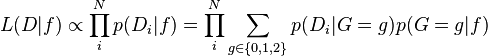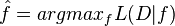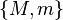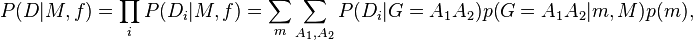ANGSD: Analysis of next generation Sequencing Data

Latest tar.gz version is (0.936/0.937 on github), see Change_log for changes, and download it here.

# SYKmaf

## ML estimator with known minor

First infer the Major and Minor allele and then use BFGS (-doMaf 1) optimazation or the EM algorithm (-doMaf 2) to estimate the allele frequencies.$L(D|f) \propto \prod_i^N p(D_i|f) = \prod_i^N \sum_{g\in\{0,1,2\}}p(D_i|G=g)p(G=g|f)$$\hat{f}=argmax_{f} L(D|f)$

## ML estimator with unknown minor

First infer the Major allele and then use BFGS (-doMaf 4) optimazation or the EM algorithm (-doMaf 8) to estimate the allele frequencies. Here only the Major allele needs to be known and the uncertaincy of infering the minor allele is modelled.

Let$\{M,m\}$ denote the major an minor allele assuming adiallelic site, then the maximum likelihood estimate of this pair is found using the likelihood function$P(D|M,f) = \prod_i P(D_i|M,f) = \sum_m \sum_{A_1,A_2} P(D_i|G=A_1A_2)p(G=A_1A_2|m,M)p(m),$